All You Need to Know About

# Excel Formulas & Functions Tutorials

Learn the must know Excel formulas & functions and become better at Excel.

## Formulas VS Functions

You most probably have heard the words Formulas & Functions both being used in Excel. What is the difference between them?
Excel Formulas are expressions which calculate the value of a cell. An Excel Function is a predefined formula that is made available for you to use in Excel:We use both terms (function and formula) interchangeably.
In our example above, the Excel Formula adds 100 to cell A1 then multiplies it by 1.1. As for our Excel Function, it uses the VLOOKUP Function to lookup for a specific value in your Excel worksheet.

## Entering Formulas in Excel

Entering Formulas in Excel is done with these simple steps:
– Select a cell in your worksheet
– You type in the equal sign, as you are telling Excel that you want to input an Excel Formula
– You can type inside cell A1, =A2 + 2
– This means you are telling Excel, get the value of A2, add 2 to it and display it in cell A1
– If you change the value of A2, the value of A1 changes too! As A1’s value is linked to A2 because of your Excel formula.
You would be mesmerized after seeing the automatic updating of the values! This is one of the coolest and most powerful features of using Formulas for Excel!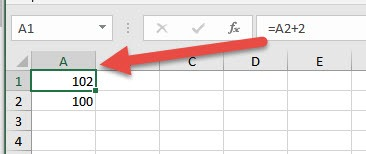## Editing Formulas in Excel

OK, now you have typed in your Excel Formulas, the question now is how do you update this if you want to make changes?
That’s easy! When you select that specific cell, Excel will show you the actual value or the formula in the formula bar at the top. Pretty much Excel will show you the actual contents that led to the value of the cell you have selected.
Now that you have the cell selected. Let us see how we can make some quick changes.
– To update your formula, click in the formula bar then make your changes to the formula.
– For example, our original formula was =A2 + 2. You can change this inside the Formula bar to =B2 + 3
– After making the changes, press Enter.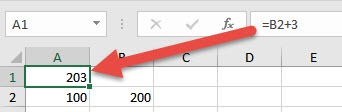## Order of Operator Precedence in Excel Formulas

Once you gain more proficiency is using formulas for Excel, your formulas start to get longer and more complex.
One important concept you need to master is the order on how calculations occur in Excel. Excel uses a default order for this. The basic rules are:
– If there is a part of your Excel formula in parenthesis, then evaluate that part first
– After that, multiplication and division operations take place, from left to right
– Then after that, addition and subtraction operations take place, from left to right as well
Let us have this example and assume the value of cell A2 is 4:
=3 * (A2 + 2 * 5)
– Since we have a part of the formula in parenthesis, we calculate that first
– We evaluate (A2 + 2 * 5) first
– Inside that group, we evaluate 2 * 5. As multiplication takes precedence over addition.
– So our formula will now look like =3 * (A2 + 10)
– Now we will evaluate (A2 + 10)
– So our formula will now look like = 3 * 14
– The rest should now be easy, multiplying these will result to 42!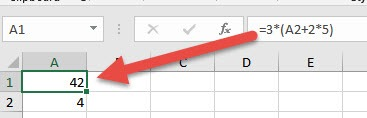Easy right? Let’s try another one and assume the value of cell A2 is still 4:
= 3 * A2 + 2 * 5
– Now this one looks exactly the same as our previous example. But without the parenthesis.
– Since we do not have any groups enclosed in parenthesis, we will evaluate the first multiplication first.
– So now we have: = 12 + 2 * 5
– Now we will evaluate the second multiplication operation.
– So now we have: = 12 + 10
– And our final result is 22!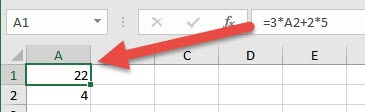## The Insert Function Wizard

The Insert Function wizard is a handy tool that you can use for inputting formulas for Excel. As there are a lot of Excel functions, it could be hard to remember what each one does and how to use them.

The general rule is every function follows the same structure. For example we have =AVERAGE(1, 2, 3). The name of the function is AVERAGE, then the parameters are what is enclosed in the parenthesis. So it’s trying to get the average of the values 1, 2, and 3.
What if you forget how to use the AVERAGE function? No problem as the Insert Function Wizard is here to the rescue!
– Let us assume you forgot how to average values in Excel. So the first step is to click the Insert function right next to the Formula Bar: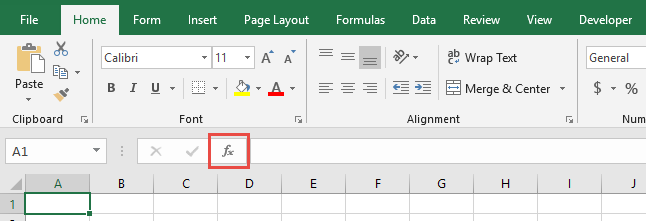– Once you have the new window, type in a short description so that this wizard will suggest the best Excel formula for you. (e.g. type in the word average)
– Now select the function that applies to you, like in our case we will select the AVERAGE function.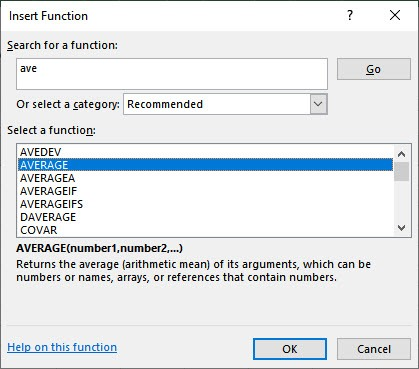– Fill in the arguments one by one depending on the function you have chosen. The cool thing is Excel provides explanations for each argument that you need to fill up with.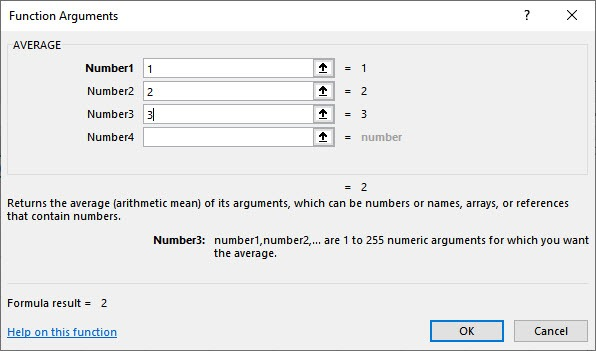– So there is no need to memorize how you would use the function as Excel provides all the necessary details for you!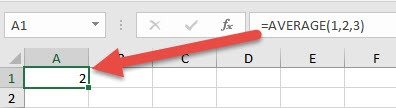To learn more about specific Formulas more in depth, we have a lot of tutorials waiting for you below!

Top 10 Tutorials

Latest Tutorials

January 25, 2023

July 6, 2021

June 7, 2021

June 4, 2021

June 4, 2021

June 1, 2021